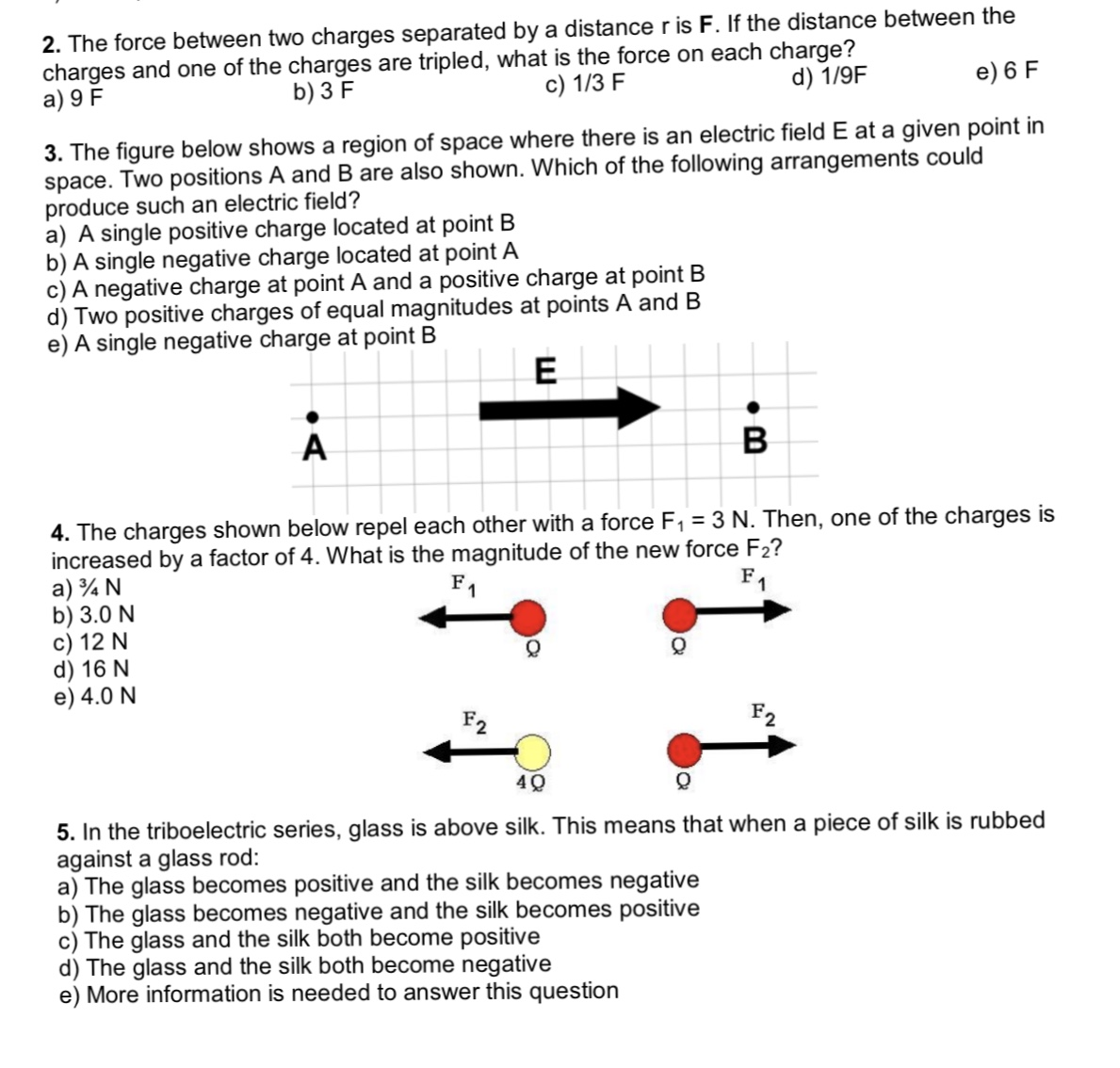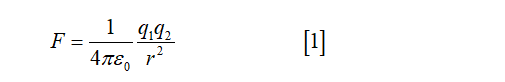# 2. The force between two charges separated by a distance r is F. If the distance between thecharges and one of the charges are tripled, what is the force on each charge?a) 9 Fb) 3 Fc) 1/3 Fd) 1/9Fe) 6 F3. The figure below shows a region of space where there is an electric field E at a given point inspace. Two positions A and B are also shown. Which of the following arrangements couldproduce such an electric field?a) A single positive charge located at point Bb) A single negative charge located at point Ac) A negative charge at point A and a positive charge at point Bd) Two positive charges of equal magnitudes at points A and Be) A single negative charge at point BB4. The charges shown below repel each other with a force F, = 3 N. Then, one of the charges isincreased by a factor of 4. What is the magnitude of the new force F2?a) ¾ Nb) 3.0 Nc) 12 Nd) 16 Ne) 4.0 NF1F1F2F25. In the triboelectric series, glass is above silk. This means thagainst a glass rod:a) The glass becomes positive and the silk becomes negativeb) The glass becomes negative and the silk becomes positivec) The glass and the silk both become positived) The glass and the silk both become negativee) More information is needed to answer this questionwhen a piece of silk is rubbed

Question
1 views

#4 with explanationhelp_outlineImage Transcriptionclose2. The force between two charges separated by a distance r is F. If the distance between the charges and one of the charges are tripled, what is the force on each charge? a) 9 F b) 3 F c) 1/3 F d) 1/9F e) 6 F 3. The figure below shows a region of space where there is an electric field E at a given point in space. Two positions A and B are also shown. Which of the following arrangements could produce such an electric field? a) A single positive charge located at point B b) A single negative charge located at point A c) A negative charge at point A and a positive charge at point B d) Two positive charges of equal magnitudes at points A and B e) A single negative charge at point B B 4. The charges shown below repel each other with a force F, = 3 N. Then, one of the charges is increased by a factor of 4. What is the magnitude of the new force F2? a) ¾ N b) 3.0 N c) 12 N d) 16 N e) 4.0 N F1 F1 F2 F2 5. In the triboelectric series, glass is above silk. This means th against a glass rod: a) The glass becomes positive and the silk becomes negative b) The glass becomes negative and the silk becomes positive c) The glass and the silk both become positive d) The glass and the silk both become negative e) More information is needed to answer this question when a piece of silk is rubbed fullscreen
check_circle

Step 1

The expression for electric force between two charges is...

### Want to see the full answer?

See Solution

#### Want to see this answer and more?

Solutions are written by subject experts who are available 24/7. Questions are typically answered within 1 hour.*

See Solution
*Response times may vary by subject and question.
Tagged in